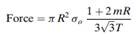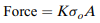### Create an Account

Home / Questions / The force required to compress a cylindrical solid between flat parallel dies see Figure 1...

# The force required to compress a cylindrical solid between flat parallel dies see Figure 16-10) has been estimated by a theory of plasticity analysis to be where R ¼ radius of the cylinder

The force required to compress a cylindrical solid between flat parallel dies (see Figure 16-10) has been estimated (by a theory of plasticity analysis) to bewhere:

R ¼ radius of the cylinder

T ¼ thickness of the cylinder

so ¼ yield strength of the material

m ¼ friction factor (between 0 and 1 where 0 is frictionless and 1 is complete sticking)

An engineering student is attempting to impress his date by demonstrating some of the neat aspects of metal forming. He places a shiny penny between the platens of a 60,000-lb capacity press and proceeds to apply pressure. Assume that the coin has a 3 4-in. diameter and is 1 16 in. thick. The yield strength is estimated as 50,000 psi, and because no lubricant is applied, friction is that of complete sticking, or m ¼ 1.0.

a. Compute the force required to induce plastic deformation.

b. If this force is greater than the capacity of the press (60,000 lb), compute the pressure when the full-capacity force of 60,000 lb is applied.

c. If the press surfaces are made from thick plates of steel with a yield strength of 120,000 psi, describe the results of the demonstration.

d. A simple model of forging force uses the equation:where:

K ¼ a dimensionless multiplying factor

so ¼ yield strength of the material

A ¼ projected area of the forging

K is assigned a value of 3–5 for simple shapes without flash, 5–8 for simple shapes with flash, and 8–12 for complex shapes with flash. Consider the two equations for forging force and discuss their similarities and differences.

May 19 2020 View more View LessSubscribe To Get Solution